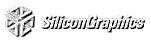# Chapter 2 Drawing Geometric Objects

Chapter Objectives

After reading this chapter, you'll be able to do the following:

Clear the window to an arbitrary color

Draw with any geometric primitive - points, lines, and polygons - in two or three dimensions

Control the display of those primitives - for example, draw dashed lines or outlined polygons

Specify normal vectors at appropriate points on the surface of solid objects

Force any pending drawing to complete

Although you can draw complex and interesting pictures using OpenGL, they're all constructed from a small number of primitive graphical items. This shouldn't be too surprising - look at what Leonardo da Vinci accomplished with just pencils and paintbrushes.

At the highest level of abstraction, there are three basic drawing operations: clearing the window, drawing a geometric object, and drawing a raster object. Raster objects, which include such things as two-dimensional images, bitmaps, and character fonts, are covered in Chapter 8 . In this chapter, you learn how to clear the screen and to draw geometric objects, including points, straight lines, and flat polygons.

You might think to yourself, "Wait a minute. I've seen lots of computer graphics in movies and on television, and there are plenty of beautifully shaded curved lines and surfaces. How are those drawn, if all OpenGL can draw are straight lines and flat polygons?" Even the image on the cover of this book includes a round table and objects on the table that have curved surfaces. It turns out that all the curved lines and surfaces you've seen are approximated by large numbers of little flat polygons or straight lines, in much the same way that the globe on the cover is constructed from a large set of rectangular blocks. The globe doesn't appear to have a smooth surface because the blocks are relatively large compared to the globe. Later in this chapter, we show you how to construct curved lines and surfaces from lots of small geometric primitives.

This chapter has the following major sections:

"A Drawing Survival Kit" explains how to clear the window and force drawing to be completed. It also gives you basic information about controlling the color of geometric objects and about hidden-surface removal.

"Describing Points, Lines, and Polygons" shows you what the set of primitive geometric objects is and how to draw them.

"Displaying Points, Lines, and Polygons" explains what control you have over the details of how primitives are drawn - for example, what diameter points have, whether lines are solid or dashed, and whether polygons are outlined or filled.

"Normal Vectors" discusses how to specify normal vectors for geometric objects and (briefly) what these vectors are for.

"Some Hints for Building Polygonal Models of Surfaces" explores the issues and techniques involved in constructing polygonal approximations to surfaces.

One thing to keep in mind as you read the rest of this chapter is that with OpenGL, unless you specify otherwise, every time you issue a drawing command, the specified object is drawn. This might seem obvious, but in some systems, you first make a list of things to draw, and when it's complete, you tell the graphics hardware to draw the items in the list. The first style is called immediate-mode graphics and is OpenGL's default style. In addition to using immediate mode, you can choose to save some commands in a list (called a display list) for later drawing. Immediate-mode graphics is typically easier to program, but display lists are often more efficient. Chapter 4 tells you how to use display lists and why you might want to use them.

## A Drawing Survival Kit

This section explains how to clear the window in preparation for drawing, set the color of objects that are to be drawn, and force drawing to be completed. None of these subjects has anything to do with geometric objects in a direct way, but any program that draws geometric objects has to deal with these issues. This section also introduces the concept of hidden-surface removal, a technique that can be used to draw geometric objects easily.

### Clearing the Window

Drawing on a computer screen is different from drawing on paper in that the paper starts out white, and all you have to do is draw the picture. On a computer, the memory holding the picture is usually filled with the last picture you drew, so you typically need to clear it to some background color before you start to draw the new scene. The color you use for the background depends on the application. For a word processor, you might clear to white (the color of the paper) before you begin to draw the text. If you're drawing a view from a spaceship, you clear to the black of space before beginning to draw the stars, planets, and alien spaceships. Sometimes you might not need to clear the screen at all; for example, if the image is the inside of a room, the entire graphics window gets covered as you draw all the walls.

At this point, you might be wondering why we keep talking about clearing the window - why not just draw a rectangle of the appropriate color that's large enough to cover the entire window? First, a special command to clear a window can be much more efficient than a general-purpose drawing command. In addition, as you'll see in Chapter 3 , OpenGL allows you to set the coordinate system, viewing position, and viewing direction arbitrarily, so it might be difficult to figure out an appropriate size and location for a window-clearing rectangle. Also, you can have OpenGL use hidden-surface removal techniques that eliminate objects obscured by others nearer to the eye; thus, if the window-clearing rectangle is to be a background, you must make sure that it's behind all the other objects of interest. With an arbitrary coordinate system and point of view, this might be difficult. Finally, on many machines, the graphics hardware consists of multiple buffers in addition to the buffer containing colors of the pixels that are displayed. These other buffers must be cleared from time to time, and it's convenient to have a single command that can clear any combination of them. (All the possible buffers are discussed in Chapter 10 .)

As an example, these lines of code clear the window to black:

```glClearColor(0.0, 0.0, 0.0, 0.0);
glClear(GL_COLOR_BUFFER_BIT);```
The first line sets the clearing color to black, and the next command clears the entire window to the current clearing color. The single parameter to glClear() indicates which buffers are to be cleared. In this case, the program clears only the color buffer, where the image displayed on the screen is kept. Typically, you set the clearing color once, early in your application, and then you clear the buffers as often as necessary. OpenGL keeps track of the current clearing color as a state variable rather than requiring you to specify it each time a buffer is cleared.

Chapter 5 and Chapter 10 talk about how other buffers are used. For now, all you need to know is that clearing them is simple. For example, to clear both the color buffer and the depth buffer, you would use the following sequence of commands:

```glClearColor(0.0, 0.0, 0.0, 0.0);
glClearDepth(0.0);
glClear(GL_COLOR_BUFFER_BIT | GL_DEPTH_BUFFER_BIT);```
In this case, the call to glClearColor() is the same as before, the glClearDepth() command specifies the value to which every pixel of the depth buffer is to be set, and the parameter to the glClear() command now consists of the logical OR of all the buffers to be cleared. The following summary of glClear() includes a table that lists the buffers that can be cleared, their names, and the chapter where each type of buffer is discussed.void glClearColor(GLclampf red, GLclampf green, GLclampf blue, GLclampf alpha);

Sets the current clearing color for use in clearing color buffers in RGBA mode. For more information on RGBA mode, see Chapter 5 . The red, green, blue, and alpha values are clamped if necessary to the range [0,1]. The default clearing color is (0, 0, 0, 0), which is black.

Clears the specified buffers to their current clearing values. The mask argument is a bitwise-ORed combination of the values listed in Table 2-1 .
Table 2-1 : Clearing Buffers
Buffer Name Reference
Color buffer GL_COLOR_BUFFER_BIT Chapter 5
Depth buffer GL_DEPTH_BUFFER_BIT Chapter 10
Accumulation buffer GL_ACCUM_BUFFER_BIT Chapter 10
Stencil buffer GL_STENCIL_BUFFER_BIT Chapter 10

Before issuing a command to clear multiple buffers, you have to set the values to which each buffer is to be cleared if you want something other than the default color, depth value, accumulation color, and stencil index. In addition to the glClearColor() and glClearDepth() commands that set the current values for clearing the color and depth buffers, glClearIndex(), glClearAccum(), and glClearStencil() specify the color index, accumulation color, and stencil index used to clear the corresponding buffers. See Chapter 5 and Chapter 10 for descriptions of these buffers and their uses.

OpenGL allows you to specify multiple buffers because clearing is generally a slow operation, since every pixel in the window (possibly millions) is touched, and some graphics hardware allows sets of buffers to be cleared simultaneously. Hardware that doesn't support simultaneous clears performs them sequentially. The difference between

`glClear(GL_COLOR_BUFFER_BIT | GL_DEPTH_BUFFER_BIT);`
and
```glClear(GL_COLOR_BUFFER_BIT);
glClear(GL_DEPTH_BUFFER_BIT);```
is that although both have the same final effect, the first example might run faster on many machines. It certainly won't run more slowly.

### Specifying a Color

With OpenGL, the description of the shape of an object being drawn is independent of the description of its color. Whenever a particular geometric object is drawn, it's drawn using the currently specified coloring scheme. The coloring scheme might be as simple as "draw everything in fire-engine red," or might be as complicated as "assume the object is made out of blue plastic, that there's a yellow spotlight pointed in such and such a direction, and that there's a general low-level reddish-brown light everywhere else." In general, an OpenGL programmer first sets the color or coloring scheme, and then draws the objects. Until the color or coloring scheme is changed, all objects are drawn in that color or using that coloring scheme. This method helps OpenGL achieve higher drawing performance than would result if it didn't keep track of the current color.

For example, the pseudocode

```set_current_color(red);
draw_object(A);
draw_object(B);
set_current_color(green);
set_current_color(blue);
draw_object(C);```
draws objects A and B in red, and object C in blue. The command on the fourth line that sets the current color to green is wasted.

Coloring, lighting, and shading are all large topics with entire chapters or large sections devoted to them. To draw geometric primitives that can be seen, however, you need some basic knowledge of how to set the current color; this information is provided in the next paragraphs. For details on these topics, see Chapter 5 and Chapter 6 .

To set a color, use the command glColor3f(). It takes three parameters, all of which are floating-point numbers between 0.0 and 1.0. The parameters are, in order, the red, green, and blue components of the color. You can think of these three values as specifying a "mix" of colors: 0.0 means don't use any of that component, and 1.0 means use all you can of that component. Thus, the code

`glColor3f(1.0, 0.0, 0.0);`
makes the brightest red the system can draw, with no green or blue components. All zeros makes black; in contrast, all ones makes white. Setting all three components to 0.5 yields gray (halfway between black and white). Here are eight commands and the colors they would set:
```glColor3f(0.0, 0.0, 0.0);                             black
glColor3f(1.0, 0.0, 0.0);                             red
glColor3f(0.0, 1.0, 0.0);                             green
glColor3f(1.0, 1.0, 0.0);                             yellow
glColor3f(0.0, 0.0, 1.0);                             blue
glColor3f(1.0, 0.0, 1.0);                             magenta
glColor3f(0.0, 1.0, 1.0);                             cyan
glColor3f(1.0, 1.0, 1.0);                             white```
You might have noticed earlier that when you're setting the color to clear the color buffer, glClearColor() takes four parameters, the first three of which match the parameters for glColor3f(). The fourth parameter is the alpha value; it's covered in detail in "Blending." For now, always set the fourth parameter to 0.0.

### Forcing Completion of Drawing

Most modern graphics systems can be thought of as an assembly line, sometimes called a graphics pipeline. The main central processing unit (CPU) issues a drawing command, perhaps other hardware does geometric transformations, clipping occurs, then shading or texturing is performed, and finally, the values are written into the bitplanes for display (see Appendix A for details on the order of operations). In high-end architectures, each of these operations is performed by a different piece of hardware that's been designed to perform its particular task quickly. In such an architecture, there's no need for the CPU to wait for each drawing command to complete before issuing the next one. While the CPU is sending a vertex down the pipeline, the transformation hardware is working on transforming the last one sent, the one before that is being clipped, and so on. In such a system, if the CPU waited for each command to complete before issuing the next, there could be a huge performance penalty.

In addition, the application might be running on more than one machine. For example, suppose that the main program is running elsewhere (on a machine called the client), and that you're viewing the results of the drawing on your workstation or terminal (the server), which is connected by a network to the client. In that case, it might be horribly inefficient to send each command over the network one at a time, since considerable overhead is often associated with each network transmission. Usually, the client gathers a collection of commands into a single network packet before sending it. Unfortunately, the network code on the client typically has no way of knowing that the graphics program is finished drawing a frame or scene. In the worst case, it waits forever for enough additional drawing commands to fill a packet, and you never see the completed drawing.

For this reason, OpenGL provides the command glFlush(), which forces the client to send the network packet even though it might not be full. Where there is no network and all commands are truly executed immediately on the server, glFlush() might have no effect. However, if you're writing a program that you want to work properly both with and without a network, include a call to glFlush() at the end of each frame or scene. Note that glFlush() doesn't wait for the drawing to complete - it just forces the drawing to begin execution, thereby guaranteeing that all previous commands execute in finite time even if no further rendering commands are executed.

A few commands - for example, commands that swap buffers in double-buffer mode - automatically flush pending commands onto the network before they can occur. void glFlush(void);

Forces previously issued OpenGL commands to begin execution, thus guaranteeing that they complete in finite time.

If glFlush() isn't sufficient for you, try glFinish(). This command flushes the network as glFlush() does and then waits for notification from the graphics hardware or network indicating that the drawing is complete in the framebuffer. You might need to use glFinish() if you want to synchronize tasks - for example, to make sure that your three-dimensional rendering is on the screen before you use Display PostScript to draw labels on top of the rendering. Another example would be to ensure that the drawing is complete before it begins to accept user input. After you issue a glFinish() command, your graphics process is blocked until it receives notification from the graphics hardware (or client, if you're running over a network) that the drawing is complete. Keep in mind that excessive use of glFinish() can reduce the performance of your application, especially if you're running over a network, because it requires round-trip communication. If glFlush() is sufficient for your needs, use it instead of glFinish().void glFinish(void);

Forces all previously issued OpenGL commands to complete. This command doesn't return until all effects from previous commands are fully realized.

### Hidden-Surface Removal Survival Kit

When you draw a scene composed of three-dimensional objects, some of them might obscure all or parts of others. Changing your viewpoint can change the obscuring relationship. For example, if you view the scene from the opposite direction, any object that was previously in front of another is now behind it. To draw a realistic scene, these obscuring relationships must be maintained. If your code works something like this
```while (1) {
get_viewing_point_from_mouse_position();
glClear(GL_COLOR_BUFFER_BIT);
draw_3d_object_A();
draw_3d_object_B();
}```
it might be that for some mouse positions, object A obscures object B, and for others, the opposite relationship might hold. If nothing special is done, the preceding code always draws object B second, and thus on top of object A, no matter what viewing position is selected.

The elimination of parts of solid objects that are obscured by others is called hidden-surface removal. (Hidden-line removal, which does the same job for objects represented as wireframe skeletons, is a bit trickier, and it isn't discussed here. See "Hidden-Line Removal," for details.) The easiest way to achieve hidden-surface removal is to use the depth buffer (sometimes called a z-buffer). (Also see Chapter 10 .)

A depth buffer works by associating a depth, or distance from the viewpoint, with each pixel on the window. Initially, the depth values for all pixels are set to the largest possible distance using the glClear() command with GL_DEPTH_BUFFER_BIT, and then the objects in the scene are drawn in any order.

Graphical calculations in hardware or software convert each surface that's drawn to a set of pixels on the window where the surface will appear if it isn't obscured by something else. In addition, the distance from the eye is computed. With depth buffering enabled, before each pixel is drawn, a comparison is done with the depth value already stored at the pixel. If the new pixel is closer to the eye than what's there, the new pixel's color and depth values replace those that are currently written into the pixel. If the new pixel's depth is greater than what's currently there, the new pixel would be obscured, and the color and depth information for the incoming pixel is discarded. Since information is discarded rather than used for drawing, hidden-surface removal can increase your performance.

To use depth buffering, you need to enable depth buffering. This has to be done only once. Each time you draw the scene, before drawing you need to clear the depth buffer and then draw the objects in the scene in any order.

To convert the preceding program fragment so that it performs hidden-surface removal, modify it to the following:

```glEnable(GL_DEPTH_TEST);
...
while (1) {
glClear(GL_COLOR_BUFFER_BIT | GL_DEPTH_BUFFER_BIT);
get_viewing_point_from_mouse_position();
draw_3d_object_A();
draw_3d_object_B(); }```
The argument to glClear() clears both the depth and color buffers.

## Describing Points, Lines, and Polygons

This section explains how to describe OpenGL geometric primitives. All geometric primitives are eventually described in terms of their vertices - coordinates that define the points themselves, the endpoints of line segments, or the corners of polygons. The next section discusses how these primitives are displayed and what control you have over their display.

### What Are Points, Lines, and Polygons?

You probably have a fairly good idea of what a mathematician means by the terms point, line, and polygon. The OpenGL meanings aren't quite the same, however, and it's important to understand the differences. The differences arise because mathematicians can think in a geometrically perfect world, whereas the rest of us have to deal with real-world limitations.

For example, one difference comes from the limitations of computer-based calculations. In any OpenGL implementation, floating-point calculations are of finite precision, and they have round-off errors. Consequently, the coordinates of OpenGL points, lines, and polygons suffer from the same problems.

Another difference arises from the limitations of a bitmapped graphics display. On such a display, the smallest displayable unit is a pixel, and although pixels might be less than 1/100th of an inch wide, they are still much larger than the mathematician's infinitely small (for points) or infinitely thin (for lines). When OpenGL performs calculations, it assumes points are represented as vectors of floating-point numbers. However, a point is typically (but not always) drawn as a single pixel, and many different points with slightly different coordinates could be drawn by OpenGL on the same pixel.

#### Points

A point is represented by a set of floating-point numbers called a vertex. All internal calculations are done as if vertices are three-dimensional. Vertices specified by the user as two-dimensional (that is, with only x and y coordinates) are assigned a z coordinate equal to zero by OpenGL.

OpenGL works in the homogeneous coordinates of three-dimensional projective geometry, so for internal calculations, all vertices are represented with four floating-point coordinates (x, y, z, w). If w is different from zero, these coordinates correspond to the euclidean three-dimensional point (x/w, y/w, z/w). You can specify the w coordinate in OpenGL commands, but that's rarely done. If the w coordinate isn't specified, it's understood to be 1.0. For more information about homogeneous coordinate systems, see Appendix G .

#### Lines

In OpenGL, line means line segment, not the mathematician's version that extends to infinity in both directions. There are easy ways to specify a connected series of line segments, or even a closed, connected series of segments (see Figure 2-1 ). In all cases, though, the lines comprising the connected series are specified in terms of the vertices at their endpoints.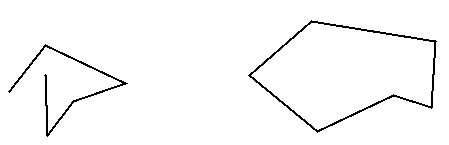Figure 2-1 : Two Connected Series of Line Segments

#### Polygons

Polygons are the areas enclosed by single closed loops of line segments, where the line segments are specified by the vertices at their endpoints. Polygons are typically drawn with the pixels in the interior filled in, but you can also draw them as outlines or a set of points, as described in "Polygon Details."

In general, polygons can be complicated, so OpenGL makes some strong restrictions on what constitutes a primitive polygon. First, the edges of OpenGL polygons can't intersect (a mathematician would call this a simple polygon). Second, OpenGL polygons must be convex, meaning that they cannot have indentations. Stated precisely, a region is convex if, given any two points in the interior, the line segment joining them is also in the interior. See Figure 2-2 for some examples of valid and invalid polygons. OpenGL, however, doesn't restrict the number of line segments making up the boundary of a convex polygon. Note that polygons with holes can't be described. They are nonconvex, and they can't be drawn with a boundary made up of a single closed loop. Be aware that if you present OpenGL with a nonconvex filled polygon, it might not draw it as you expect. For instance, on most systems no more than the convex hull of the polygon would be filled, but on some systems, less than the convex hull might be filled.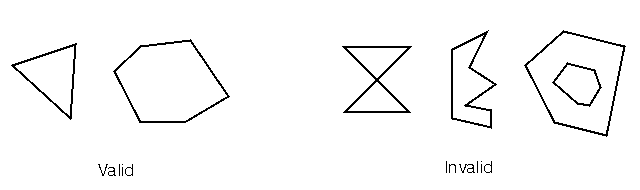Figure 2-2 : Valid and Invalid Polygons

For many applications, you need nonsimple polygons, nonconvex polygons, or polygons with holes. Since all such polygons can be formed from unions of simple convex polygons, some routines to describe more complex objects are provided in the GLU. These routines take complex descriptions and tessellate them, or break them down into groups of the simpler OpenGL polygons that can then be rendered. (See Appendix C for more information about the tessellation routines.) The reason for OpenGL's restrictions on valid polygon types is that it's simpler to provide fast polygon-rendering hardware for that restricted class of polygons.

Since OpenGL vertices are always three-dimensional, the points forming the boundary of a particular polygon don't necessarily lie on the same plane in space. (Of course, they do in many cases - if all the z coordinates are zero, for example, or if the polygon is a triangle.) If a polygon's vertices don't lie in the same plane, then after various rotations in space, changes in the viewpoint, and projection onto the display screen, the points might no longer form a simple convex polygon. For example, imagine a four-point quadrilateral where the points are slightly out of plane, and look at it almost edge-on. You can get a nonsimple polygon that resembles a bow tie, as shown in Figure 2-3 , which isn't guaranteed to render correctly. This situation isn't all that unusual if you approximate surfaces by quadrilaterals made of points lying on the true surface. You can always avoid the problem by using triangles, since any three points always lie on a plane.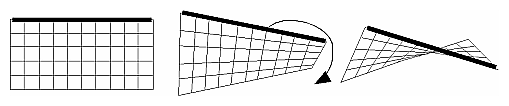Figure 2-3 : Nonplanar Polygon Transformed to Nonsimple Polygon

#### Rectangles

Since rectangles are so common in graphics applications, OpenGL provides a filled-rectangle drawing primitive, glRect*(). You can draw a rectangle as a polygon, as described in "OpenGL Geometric Drawing Primitives," but your particular implementation of OpenGL might have optimized glRect*() for rectangles.void glRect{sifd}(TYPEx1, TYPEy1, TYPEx2, TYPEy2);
void glRect{sifd}v(TYPE*v1, TYPE*v2);

Draws the rectangle defined by the corner points (x1, y1) and (x2, y2). The rectangle lies in the plane z=0 and has sides parallel to the x- and y-axes. If the vector form of the function is used, the corners are given by two pointers to arrays, each of which contains an (x, y) pair.

Note that although the rectangle begins with a particular orientation in three-dimensional space (in the x-y plane and parallel to the axes), you can change this by applying rotations or other transformations. See Chapter 3 for information about how to do this.

#### Curves

Any smoothly curved line or surface can be approximated - to any arbitrary degree of accuracy - by short line segments or small polygonal regions. Thus, subdividing curved lines and surfaces sufficiently and then approximating them with straight line segments or flat polygons makes them appear curved (see Figure 2-4 ). If you're skeptical that this really works, imagine subdividing until each line segment or polygon is so tiny that it's smaller than a pixel on the screen.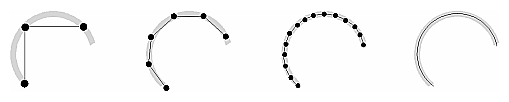Figure 2-4 : Approximating Curves

Even though curves aren't geometric primitives, OpenGL does provide some direct support for drawing them. See Chapter 11 for information about how to draw curves and curved surfaces.

### Specifying Vertices

With OpenGL, all geometric objects are ultimately described as an ordered set of vertices. You use the glVertex*() command to specify a vertex. void glVertex{234}{sifd}[v](TYPEcoords);

Specifies a vertex for use in describing a geometric object. You can supply up to four coordinates (x, y, z, w) for a particular vertex or as few as two (x, y) by selecting the appropriate version of the command. If you use a version that doesn't explicitly specify z or w, z is understood to be 0 and w is understood to be 1. Calls to glVertex*() should be executed between a glBegin() and glEnd() pair.

Here are some examples of using glVertex*():

```glVertex2s(2, 3);
glVertex3d(0.0, 0.0, 3.1415926535898);
glVertex4f(2.3, 1.0, -2.2, 2.0);

GLdouble dvect = {5.0, 9.0, 1992.0};
glVertex3dv(dvect);```
The first example represents a vertex with three-dimensional coordinates (2, 3, 0). (Remember that if it isn't specified, the z coordinate is understood to be 0.) The coordinates in the second example are (0.0, 0.0, 3.1415926535898) (double-precision floating-point numbers). The third example represents the vertex with three-dimensional coordinates (1.15, 0.5, -1.1). (Remember that the x, y, and z coordinates are eventually divided by the w coordinate.) In the final example, dvect is a pointer to an array of three double-precision floating-point numbers.

On some machines, the vector form of glVertex*() is more efficient, since only a single parameter needs to be passed to the graphics subsystem, and special hardware might be able to send a whole series of coordinates in a single batch. If your machine is like this, it's to your advantage to arrange your data so that the vertex coordinates are packed sequentially in memory.

### OpenGL Geometric Drawing Primitives

Now that you've seen how to specify vertices, you still need to know how to tell OpenGL to create a set of points, a line, or a polygon from those vertices. To do this, you bracket each set of vertices between a call to glBegin() and a call to glEnd(). The argument passed to glBegin() determines what sort of geometric primitive is constructed from the vertices. For example, the following code specifies the vertices for the polygon shown in Figure 2-5 :
```glBegin(GL_POLYGON);
glVertex2f(0.0, 0.0);
glVertex2f(0.0, 3.0);
glVertex2f(3.0, 3.0);
glVertex2f(4.0, 1.5);
glVertex2f(3.0, 0.0);
glEnd();```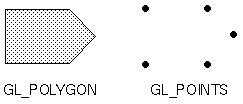Figure 2-5 : Drawing a Polygon or a Set of Points

If you had used GL_POINTS instead of GL_POLYGON, the primitive would have been simply the five points shown in Figure 2-5 . Table 2-2 in the following function summary for glBegin() lists the ten possible arguments and the corresponding type of primitive.void glBegin(GLenum mode);

Marks the beginning of a vertex list that describes a geometric primitive. The type of primitive is indicated by mode, which can be any of the values shown in Table 2-2 .
Table 2-2 : Geometric Primitive Names and Meanings
Value Meaning
GL_POINTS individual points
GL_LINES pairs of vertices interpreted as individual line segments
GL_POLYGON boundary of a simple, convex polygon
GL_TRIANGLES triples of vertices interpreted as triangles
GL_QUADS quadruples of vertices interpreted as four-sided polygons
GL_LINE_STRIP series of connected line segments
GL_LINE_LOOP same as above, with a segment added between last and first vertices
GL_TRIANGLE_STRIP linked strip of triangles
GL_TRIANGLE_FAN linked fan of triangles

void glEnd(void);

Marks the end of a vertex list.

Figure 2-6 shows examples of all the geometric primitives listed in Table 2-2 . The paragraphs that follow the figure give precise descriptions of the pixels that are drawn for each of the objects. Note that in addition to points, several types of lines and polygons are defined. Obviously, you can find many ways to draw the same primitive. The method you choose depends on your vertex data.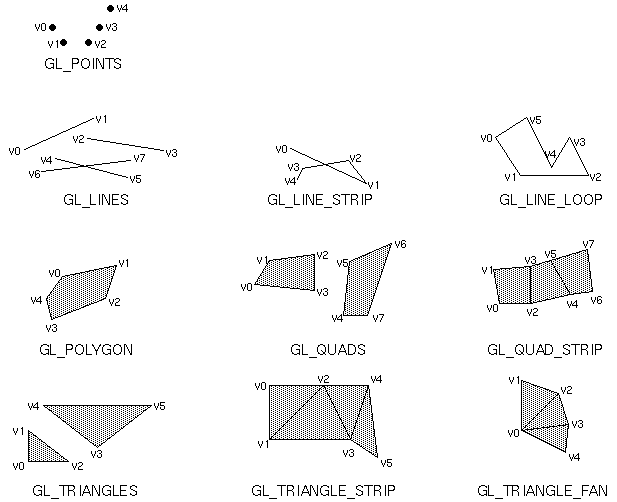Figure 2-6 : Geometric Primitive Types

As you read the following descriptions, assume that n vertices (v0, v1, v2, ... , vn-1) are described between a glBegin() and glEnd() pair.

GL_POINTS

Draws a point at each of the n vertices.
GL_LINES
Draws a series of unconnected line segments. Segments are drawn between v0 and v1, between v2 and v3, and so on. If n is odd, the last segment is drawn between vn-3 and vn-2, and vn-1 is ignored.
GL_POLYGON
Draws a polygon using the points v0, ... , vn-1 as vertices. n must be at least 3, or nothing is drawn. In addition, the polygon specified must not intersect itself and must be convex. If the vertices don't satisfy these conditions, the results are unpredictable.
GL_TRIANGLES
Draws a series of triangles (three-sided polygons) using vertices v0, v1, v2, then v3, v4, v5, and so on. If n isn't an exact multiple of 3, the final one or two vertices are ignored.
GL_LINE_STRIP
Draws a line segment from v0 to v1, then from v1 to v2, and so on, finally drawing the segment from vn-2 to vn-1. Thus, a total of n-1 line segments are drawn. Nothing is drawn unless n is larger than 1. There are no restrictions on the vertices describing a line strip (or a line loop); the lines can intersect arbitrarily.
GL_LINE_LOOP
Same as GL_LINE_STRIP, except that a final line segment is drawn from vn-1 to v0, completing a loop.
Draws a series of quadrilaterals (four-sided polygons) using vertices v0, v1, v2, v3, then v4, v5, v6, v7, and so on. If n isn't a multiple of 4, the final one, two, or three vertices are ignored.
Draws a series of quadrilaterals (four-sided polygons) beginning with v0, v1, v3, v2, then v2, v3, v5, v4, then v4, v5, v7, v6, and so on. See Figure 2-6 . n must be at least 4 before anything is drawn, and if n is odd, the final vertex is ignored.
GL_TRIANGLE_STRIP
Draws a series of triangles (three-sided polygons) using vertices v0, v1, v2, then v2, v1, v3 (note the order), then v2, v3, v4, and so on. The ordering is to ensure that the triangles are all drawn with the same orientation so that the strip can correctly form part of a surface. Figure 2-6 should make the reason for the ordering obvious. n must be at least 3 for anything to be drawn.
GL_TRIANGLE_FAN
Same as GL_TRIANGLE_STRIP, except that the vertices are v0, v1, v2, then v0, v2, v3, then v0, v3, v4, and so on. Look at Figure 2-6 .

#### Restrictions on Using glBegin() and glEnd()

The most important information about vertices is their coordinates, which are specified by the glVertex*() command. You can also supply additional vertex-specific data for each vertex - a color, a normal vector, texture coordinates, or any combination of these - using special commands. In addition, a few other commands are valid between a glBegin() and glEnd() pair. Table 2-3 contains a complete list of such valid commands.
Table 2-3 : Valid Commands between glBegin() and glEnd()
Command Purpose of Command Reference
glVertex*() set vertex coordinates Chapter 2
glColor*() set current color Chapter 5
glIndex*() set current color index Chapter 5
glNormal*() set normal vector coordinates Chapter 2
glEvalCoord*() generate coordinates Chapter 11
glCallList(), glCallLists() execute display list(s) Chapter 4
glTexCoord*() set texture coordinates Chapter 9
glEdgeFlag*() control drawing of edges Chapter 2
glMaterial*() set material properties Chapter 6

No other OpenGL commands are valid between a glBegin() and glEnd() pair, and making any other OpenGL call generates an error. Note, however, that only OpenGL commands are restricted; you can certainly include other programming-language constructs. For example, the following code draws an outlined circle:

```#define PI 3.1415926535897;
GLint circle_points = 100;
glBegin(GL_LINE_LOOP);
for (i = 0; i < circle_points; i++) {
angle = 2*PI*i/circle_points;
glVertex2f(cos(angle), sin(angle));
}
glEnd();```
This example isn't the most efficient way to draw a circle, especially if you intend to do it repeatedly. The graphics commands used are typically very fast, but this code calculates an angle and calls the sin() and cos() routines for each vertex; in addition, there's the loop overhead. If you need to draw lots of circles, calculate the coordinates of the vertices once and save them in an array, create a display list (see Chapter 4 ,) or use a GLU routine (see Appendix C .)

Unless they are being compiled into a display list, all glVertex*() commands should appear between some glBegin() and glEnd() combination. (If they appear elsewhere, they don't accomplish anything.) If they appear in a display list, they are executed only if they appear between a glBegin() and a glEnd().

Although many commands are allowed between glBegin() and glEnd(), vertices are generated only when a glVertex*() command is issued. At the moment glVertex*() is called, OpenGL assigns the resulting vertex the current color, texture coordinates, normal vector information, and so on. To see this, look at the following code sequence. The first point is drawn in red, and the second and third ones in blue, despite the extra color commands:

```glBegin(GL_POINTS);
glColor3f(0.0, 1.0, 0.0);                  /* green */
glColor3f(1.0, 0.0, 0.0);                  /* red */
glVertex(...);
glColor3f(1.0, 1.0, 0.0);                  /* yellow */
glColor3f(0.0, 0.0, 1.0);                  /* blue */
glVertex(...);
glVertex(...);
glEnd();```
You can use any combination of the twenty-four versions of the glVertex*() command between glBegin() and glEnd(), although in real applications all the calls in any particular instance tend to be of the same form.

## Displaying Points, Lines, and Polygons

By default, a point is drawn as a single pixel on the screen, a line is drawn solid and one pixel wide, and polygons are drawn solidly filled in. The following paragraphs discuss the details of how to change these default display modes.

### Point Details

To control the size of a rendered point, use glPointSize() and supply the desired size in pixels as the argument. void glPointSize(GLfloat size);

Sets the width in pixels for rendered points; size must be greater than 0.0 and by default is 1.0.

The actual collection of pixels on the screen that are drawn for various point widths depends on whether antialiasing is enabled. (Antialiasing is a technique for smoothing points and lines as they're rendered. This topic is covered in detail in "Antialiasing." ) If antialiasing is disabled (the default), fractional widths are rounded to integer widths, and a screen-aligned square region of pixels is drawn. Thus, if the width is 1.0, the square is one pixel by one pixel; if the width is 2.0, the square is two pixels by two pixels, and so on.

With antialiasing enabled, a circular group of pixels is drawn, and the pixels on the boundaries are typically drawn at less than full intensity to give the edge a smoother appearance. In this mode, nonintegral widths aren't rounded.

Most OpenGL implementations support very large point sizes. A particular implementation, however, might limit the size of nonantialiased points to its maximum antialiased point size, rounded to the nearest integer value. You can obtain this floating-point value by using GL_POINT_SIZE_RANGE with glGetFloatv().

### Line Details

With OpenGL, you can specify lines with different widths and lines that are stippled in various ways - dotted, dashed, drawn with alternating dots and dashes, and so on.

#### Wide Lines

void glLineWidth(GLfloat width);

Sets the width in pixels for rendered lines; width must be greater than 0.0 and by default is 1.0.

The actual rendering of lines is affected by the antialiasing mode, in the same way as for points. (See "Antialiasing." ) Without antialiasing, widths of 1, 2, and 3 draw lines one, two, and three pixels wide. With antialiasing enabled, nonintegral line widths are possible, and pixels on the boundaries are typically partially filled. As with point sizes, a particular OpenGL implementation might limit the width of nonantialiased lines to its maximum antialiased line width, rounded to the nearest integer value. You can obtain this floating-point value by using GL_LINE_WIDTH_RANGE with glGetFloatv().

Keep in mind that by default lines are one pixel wide, so they appear wider on lower-resolution screens. For computer displays, this isn't typically an issue, but if you're using OpenGL to render to a high-resolution plotter, one-pixel lines might be nearly invisible. To obtain resolution-independent line widths, you need to take into account the physical dimensions of pixels.

With nonantialiased wide lines, the line width isn't measured perpendicular to the line. Instead, it's measured in the y direction if the absolute value of the slope is less than 1.0; otherwise, it's measured in the x direction. The rendering of an antialiased line is exactly equivalent to the rendering of a filled rectangle of the given width, centered on the exact line. See "Polygon Details," for a discussion of the rendering of filled polygonal regions.

#### Stippled Lines

To make stippled (dotted or dashed) lines, you use the command glLineStipple() to define the stipple pattern, and then you enable line stippling with glEnable():
```glLineStipple(1, 0x3F07);
glEnable(GL_LINE_STIPPLE);```
void glLineStipple(GLint factor, GLushort pattern);

Sets the current stippling pattern for lines. The pattern argument is a 16-bit series of 0s and 1s, and it's repeated as necessary to stipple a given line. A 1 indicates that drawing occurs, and 0 that it does not, on a pixel-by-pixel basis, beginning with the low-order bits of the pattern. The pattern can be stretched out by using factor, which multiplies each subseries of consecutive 1s and 0s. Thus, if three consecutive 1s appear in the pattern, they're stretched to six if factor is 2. factor is clamped to lie between 1 and 255. Line stippling must be enabled by passing GL_LINE_STIPPLE to glEnable(); it's disabled by passing the same argument to glDisable().

With the preceding example and the pattern 0x3F07 (which translates to 0011111100000111 in binary), a line would be drawn with 3 pixels on, then 5 off, 6 on, and 2 off. (If this seems backward, remember that the low-order bits are used first.) If factor had been 2, the pattern would have been elongated: 6 pixels on, 10 off, 12 on, and 4 off. Figure 2-7 shows lines drawn with different patterns and repeat factors. If you don't enable line stippling, drawing proceeds as if pattern were 0xFFFF and factor 1. (Use glDisable() with GL_LINE_STIPPLE to disable stippling.) Note that stippling can be used in combination with wide lines to produce wide stippled lines.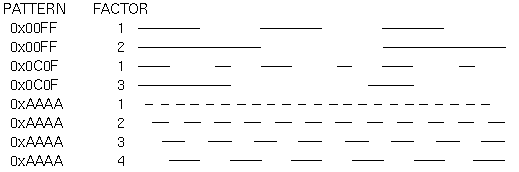Figure 2-7 : Stippled Lines

One way to think of the stippling is that as the line is being drawn, the pattern is shifted by one bit each time a pixel is drawn (or factor pixels are drawn, if factor isn't 1). When a series of connected line segments is drawn between a single glBegin() and glEnd(), the pattern continues to shift as one segment turns into the next. This way, a stippling pattern continues across a series of connected line segments. When glEnd() is executed, the pattern is reset, and - if more lines are drawn before stippling is disabled - the stippling restarts at the beginning of the pattern. If you're drawing lines with GL_LINES, the pattern resets for each independent line.

Example 2-1 illustrates the results of drawing with a couple of different stipple patterns and line widths. It also illustrates what happens if the lines are drawn as a series of individual segments instead of a single connected line strip. The results of running the program appear in Figure 2-8 .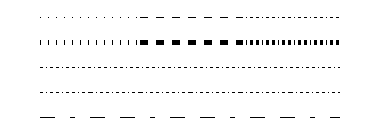Figure 2-8 : Wide Stippled Lines

Example 2-1 : Using Line Stipple Patterns: lines.c

```#include <GL/gl.h>
#include <GL/glu.h>
#include "aux.h"

#define   drawOneLine(x1,y1,x2,y2) glBegin(GL_LINES); \
glVertex2f ((x1),(y1)); glVertex2f ((x2),(y2)); glEnd();

void myinit (void) {
/*  background to be cleared to black   */
glClearColor (0.0, 0.0, 0.0, 0.0);
}

void display(void)
{
int i;

glClear (GL_COLOR_BUFFER_BIT);
/*  draw all lines in white   */
glColor3f (1.0, 1.0, 1.0);

/*  in 1st row, 3 lines, each with a different stipple   */
glEnable (GL_LINE_STIPPLE);
glLineStipple (1, 0x0101);   /*  dotted   */
drawOneLine (50.0, 125.0, 150.0, 125.0);
glLineStipple (1, 0x00FF);   /*  dashed   */
drawOneLine (150.0, 125.0, 250.0, 125.0);
glLineStipple (1, 0x1C47);   /*  dash/dot/dash   */
drawOneLine (250.0, 125.0, 350.0, 125.0);

/*  in 2nd row, 3 wide lines, each with different stipple */
glLineWidth (5.0);
glLineStipple (1, 0x0101);
drawOneLine (50.0, 100.0, 150.0, 100.0);
glLineStipple (1, 0x00FF);
drawOneLine (150.0, 100.0, 250.0, 100.0);
glLineStipple (1, 0x1C47);
drawOneLine (250.0, 100.0, 350.0, 100.0);
glLineWidth (1.0);

/*  in 3rd row, 6 lines, with dash/dot/dash stipple,   */
/*  as part of a single connected line strip         */
glLineStipple (1, 0x1C47);
glBegin (GL_LINE_STRIP);
for (i = 0; i < 7; i++)
glVertex2f (50.0 + ((GLfloat) i * 50.0), 75.0);
glEnd ();

/*  in 4th row, 6 independent lines,   */
/*  with dash/dot/dash stipple         */
for (i = 0; i < 6; i++) {
drawOneLine (50.0 + ((GLfloat) i * 50.0),
50.0, 50.0 + ((GLfloat)(i+1) * 50.0), 50.0);
}

/*  in 5th row, 1 line, with dash/dot/dash stipple   */
/*  and repeat factor of 5         */
glLineStipple (5, 0x1C47);
drawOneLine (50.0, 25.0, 350.0, 25.0);
glFlush ();
}

int main(int argc, char** argv)
{
auxInitDisplayMode (AUX_SINGLE | AUX_RGBA);
auxInitPosition (0, 0, 400, 150);
auxInitWindow (argv);
myinit ();
auxMainLoop(display);
}```

### Polygon Details

Polygons are typically drawn by filling in all the pixels enclosed within the boundary, but you can also draw them as outlined polygons, or simply as points at the vertices. A filled polygon might be solidly filled, or stippled with a certain pattern. Although the exact details are omitted here, polygons are drawn in such a way that if adjacent polygons share an edge or vertex, the pixels making up the edge or vertex are drawn exactly once - they're included in only one of the polygons. This is done so that partially transparent polygons don't have their edges drawn twice, which would make those edges appear darker (or brighter, depending on what color you're drawing with). Note that it might result in narrow polygons having no filled pixels in one or more rows or columns of pixels. Antialiasing polygons is more complicated than for points and lines; see "Antialiasing," for details.

#### Polygons as Points, Outlines, or Solids

A polygon has two sides - front and back - and might be rendered differently depending on which side is facing the viewer. This allows you to have cutaway views of solid objects in which there is an obvious distinction between the parts that are inside and those that are outside. By default, both front and back faces are drawn in the same way. To change this, or to draw only outlines or vertices, use glPolygonMode(). void glPolygonMode(GLenum face, GLenum mode);

Controls the drawing mode for a polygon's front and back faces. The parameter face can be GL_FRONT_AND_BACK, GL_FRONT, or GL_BACK; mode can be GL_POINT, GL_LINE, or GL_FILL to indicate whether the polygon should be drawn as points, outlined, or filled. By default, both the front and back faces are drawn filled.

For example, you can have the front faces filled and the back faces outlined with two calls to this routine:

```glPolygonMode(GL_FRONT, GL_FILL);
glPolygonMode(GL_BACK, GL_LINE);```
See the next section for more information about how to control which faces are considered front-facing and which back-facing.

#### Reversing and Culling Polygon Faces

By convention, polygons whose vertices appear in counterclockwise order on the screen are called front-facing. You can construct the surface of any "reasonable" solid - a mathematician would call such a surface an orientable manifold (spheres, donuts, and teapots are orientable; Klein bottles and Möbius strips aren't) - from polygons of consistent orientation. In other words, you can use all clockwise polygons, or all counterclockwise polygons. (This is essentially the mathematical definition of orientable.)

Suppose you've consistently described a model of an orientable surface but that you happen to have the clockwise orientation on the outside. You can swap what OpenGL considers the back face by using the function glFrontFace(), supplying the desired orientation for front-facing polygons.void glFrontFace(GLenum mode);

Controls how front-facing polygons are determined. By default, mode is GL_CCW, which corresponds to a counterclockwise orientation of the ordered vertices of a projected polygon in window coordinates. If mode is GL_CW, faces with a clockwise orientation are considered front-facing.

In more technical terms, the decision of whether a face of a polygon is front- or back-facing depends on the sign of the polygon's area computed in window coordinates. One way to compute this area is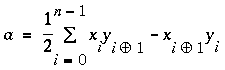where xi and yi are the x and y window coordinates of the ith vertex of the n-vertex polygon and: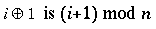Assuming that GL_CCW has been specified, if a>0, the polygon corresponding to that vertex is considered to be front-facing; otherwise, it's back-facing. If GL_CW is specified and if a<0, then the corresponding polygon is front-facing; otherwise, it's back-facing.

In a completely enclosed surface constructed from polygons with a consistent orientation, none of the back-facing polygons are ever visible - they're always obscured by the front-facing polygons. In this situation, you can maximize drawing speed by having OpenGL discard polygons as soon as it determines that they're back-facing. Similarly, if you are inside the object, only back-facing polygons are visible. To instruct OpenGL to discard front- or back-facing polygons, use the command glCullFace() and enable culling with glEnable(). void glCullFace(GLenum mode);

Indicates which polygons should be discarded (culled) before they're converted to screen coordinates. The mode is either GL_FRONT, GL_BACK, or GL_FRONT_AND_BACK to indicate front-facing, back-facing, or all polygons. To take effect, culling must be enabled using glEnable() with GL_CULL_FACE; it can be disabled with glDisable() and the same argument.

#### Stippling Polygons

By default, filled polygons are drawn with a solid pattern. They can also be filled with a 32-bit by 32-bit window-aligned stipple pattern, which you specify with glPolygonStipple(). void glPolygonStipple(const GLubyte *mask);

Defines the current stipple pattern for filled polygons. The argument mask is a pointer to a 32 × 32 bitmap that's interpreted as a mask of 0s and 1s. Where a 1 appears, the corresponding pixel in the polygon is drawn, and where a 0 appears, nothing is drawn. Figure 2-9 shows how a stipple pattern is constructed from the characters in mask. Polygon stippling is enabled and disabled by using glEnable() and glDisable() with GL_POLYGON_STIPPLE as the argument. The interpretation of the mask data is affected by the glPixelStore*() GL_UNPACK* modes. See "Controlling Pixel-Storage Modes."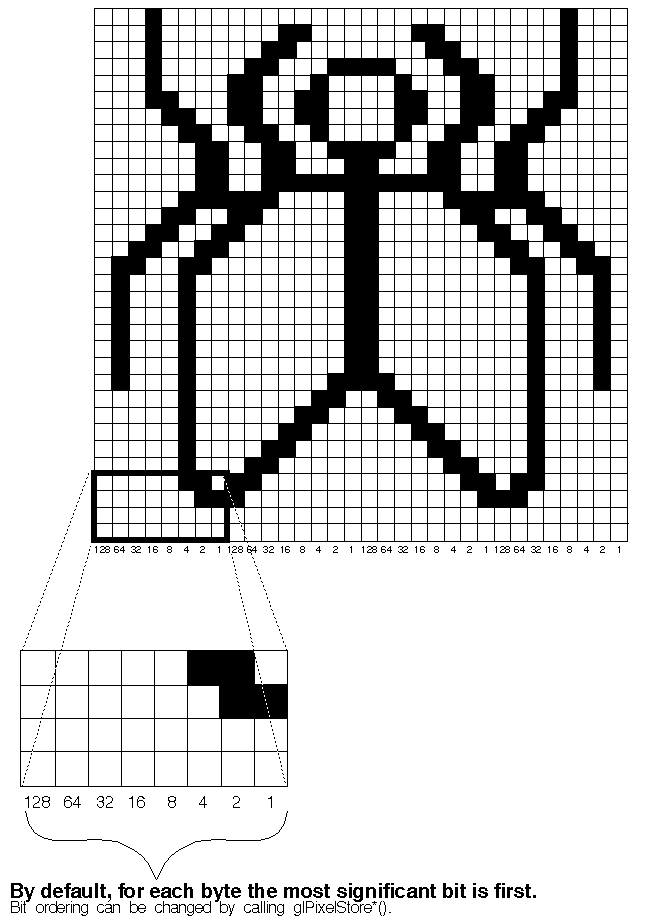Figure 2-9 : Constructing a Polygon Stipple Pattern

In addition to defining the current polygon stippling pattern, you must enable stippling:

`glEnable(GL_POLYGON_STIPPLE);`
Use glDisable() with the same argument to disable polygon stippling.

Figure 2-10 shows the results of polygons drawn unstippled and then with two different stippling patterns. The program is shown in Example 2-2 . The reversal of white to black (from Figure 2-9 to Figure 2-10 ) occurs because the program draws in white over a black background, using the pattern in Figure 2-9 as a stencil.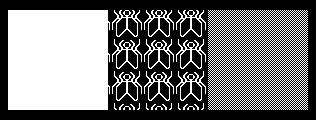Figure 2-10 : Stippled Polygons

Example 2-2 : Using Polygon Stipple Patterns: polys.c

```#include <GL/gl.h>
#include <GL/glu.h>
#include "aux.h"

void display(void)
{
GLubyte fly[] = {
0x00, 0x00, 0x00, 0x00, 0x00, 0x00, 0x00, 0x00,
0x03, 0x80, 0x01, 0xC0, 0x06, 0xC0, 0x03, 0x60,
0x04, 0x60, 0x06, 0x20, 0x04, 0x30, 0x0C, 0x20,
0x04, 0x18, 0x18, 0x20, 0x04, 0x0C, 0x30, 0x20,
0x04, 0x06, 0x60, 0x20, 0x44, 0x03, 0xC0, 0x22,
0x44, 0x01, 0x80, 0x22, 0x44, 0x01, 0x80, 0x22,
0x44, 0x01, 0x80, 0x22, 0x44, 0x01, 0x80, 0x22,
0x44, 0x01, 0x80, 0x22, 0x44, 0x01, 0x80, 0x22,
0x66, 0x01, 0x80, 0x66, 0x33, 0x01, 0x80, 0xCC,
0x19, 0x81, 0x81, 0x98, 0x0C, 0xC1, 0x83, 0x30,
0x07, 0xe1, 0x87, 0xe0, 0x03, 0x3f, 0xfc, 0xc0,
0x03, 0x31, 0x8c, 0xc0, 0x03, 0x33, 0xcc, 0xc0,
0x06, 0x64, 0x26, 0x60, 0x0c, 0xcc, 0x33, 0x30,
0x18, 0xcc, 0x33, 0x18, 0x10, 0xc4, 0x23, 0x08,
0x10, 0x63, 0xC6, 0x08, 0x10, 0x30, 0x0c, 0x08,
0x10, 0x18, 0x18, 0x08, 0x10, 0x00, 0x00, 0x08};

GLubyte halftone[] = {
0xAA, 0xAA, 0xAA, 0xAA, 0x55, 0x55, 0x55, 0x55,
0xAA, 0xAA, 0xAA, 0xAA, 0x55, 0x55, 0x55, 0x55,
0xAA, 0xAA, 0xAA, 0xAA, 0x55, 0x55, 0x55, 0x55,
0xAA, 0xAA, 0xAA, 0xAA, 0x55, 0x55, 0x55, 0x55,
0xAA, 0xAA, 0xAA, 0xAA, 0x55, 0x55, 0x55, 0x55,
0xAA, 0xAA, 0xAA, 0xAA, 0x55, 0x55, 0x55, 0x55,
0xAA, 0xAA, 0xAA, 0xAA, 0x55, 0x55, 0x55, 0x55,
0xAA, 0xAA, 0xAA, 0xAA, 0x55, 0x55, 0x55, 0x55,
0xAA, 0xAA, 0xAA, 0xAA, 0x55, 0x55, 0x55, 0x55,
0xAA, 0xAA, 0xAA, 0xAA, 0x55, 0x55, 0x55, 0x55,
0xAA, 0xAA, 0xAA, 0xAA, 0x55, 0x55, 0x55, 0x55,
0xAA, 0xAA, 0xAA, 0xAA, 0x55, 0x55, 0x55, 0x55,
0xAA, 0xAA, 0xAA, 0xAA, 0x55, 0x55, 0x55, 0x55,
0xAA, 0xAA, 0xAA, 0xAA, 0x55, 0x55, 0x55, 0x55,
0xAA, 0xAA, 0xAA, 0xAA, 0x55, 0x55, 0x55, 0x55,
0xAA, 0xAA, 0xAA, 0xAA, 0x55, 0x55, 0x55, 0x55};

glClear (GL_COLOR_BUFFER_BIT);

glColor3f (1.0, 1.0, 1.0);

glRectf (25.0, 25.0, 125.0, 125.0);
glEnable (GL_POLYGON_STIPPLE);
glPolygonStipple (fly);
glRectf (125.0, 25.0, 225.0, 125.0);
glPolygonStipple (halftone);
glRectf (225.0, 25.0, 325.0, 125.0);
glDisable (GL_POLYGON_STIPPLE);

glFlush ();
}

void myinit (void)
{
glClearColor (0.0, 0.0, 0.0, 0.0);
}

int main(int argc, char** argv)
{
auxInitDisplayMode (AUX_SINGLE | AUX_RGBA);
auxInitPosition (0, 0, 350, 150);
auxInitWindow (argv);
myinit ();
auxMainLoop(display);
}```
As mentioned in "Display-List Design Philosophy," you might want to use display lists to store polygon stipple patterns to maximize efficiency.

#### Marking Polygon Boundary Edges

OpenGL can render only convex polygons, but many nonconvex polygons arise in practice. To draw these nonconvex polygons, you typically subdivide them into convex polygons - usually triangles, as shown in Figure 2-11 - and then draw the triangles. Unfortunately, if you decompose a general polygon into triangles and draw the triangles, you can't really use glPolygonMode() to draw the polygon's outline, since you get all the triangle outlines inside it. To solve this problem, you can tell OpenGL whether a particular vertex precedes a boundary edge; OpenGL keeps track of this information by passing along with each vertex a bit indicating whether that vertex is followed by a boundary edge. Then, when a polygon is drawn in GL_LINE mode, the nonboundary edges aren't drawn. In Figure 2-11 , the dashed lines represent added edges.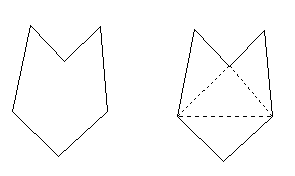Figure 2-11 : Subdividing a Nonconvex Polygon

By default, all vertices are marked as preceding a boundary edge, but you can manually control the setting of the edge flag with the command glEdgeFlag*(). This command is used between glBegin() and glEnd() pairs, and it affects all the vertices specified after it until the next glEdgeFlag() call is made. It applies only to vertices specified for polygons, triangles, and quads, not to those specified for strips of triangles or quads. void glEdgeFlag(GLboolean flag);
void glEdgeFlagv(const GLboolean *flag);

Indicates whether a vertex should be considered as initializing a boundary edge of a polygon. If flag is GL_TRUE, the edge flag is set to TRUE (the default), and any vertices created are considered to precede boundary edges until this function is called again with flag being 0.

As an example, Example 2-3 draws the outline shown in Figure 2-12 .Figure 2-12 : An Outlined Polygon Drawn Using Edge Flags

Example 2-3 : Marking Polygon Boundary Edges

```glPolygonMode(GL_FRONT_AND_BACK, GL_LINE);
glBegin(GL_POLYGON);
glEdgeFlag(GL_TRUE);
glVertex3fv(V0);
glEdgeFlag(GL_FALSE);
glVertex3fv(V1);
glEdgeFlag(GL_TRUE);
glVertex3fv(V2);
glEnd();```

## Normal Vectors

A normal vector (or normal, for short) is a vector that points in a direction that's perpendicular to a surface. For a flat surface, one perpendicular direction suffices for every point on the surface, but for a general curved surface, the normal direction might be different at each point. With OpenGL, you can specify a normal for each vertex. Vertices might share the same normal, but you can't assign normals anywhere other than at the vertices.

An object's normal vectors define the orientation of its surface in space - in particular, its orientation relative to light sources. These vectors are used by OpenGL to determine how much light the object receives at its vertices. Lighting - a large topic by itself - is the subject of Chapter 6 , and you might want to review the following information after you've read that chapter. Normal vectors are discussed briefly here because you generally define normal vectors for an object at the same time you define the object's geometry.

You use glNormal*() to set the current normal to the value of the argument passed in. Subsequent calls to glVertex*() cause the specified vertices to be assigned the current normal. Often, each vertex has a different normal, which necessitates a series of alternating calls like this:

```glBegin (GL_POLYGON);
glNormal3fv(n0);
glVertex3fv(v0);
glNormal3fv(n1);
glVertex3fv(v1);
glNormal3fv(n2);
glVertex3fv(v2);
glNormal3fv(n3);
glVertex3fv(v3);
glEnd();```
void glNormal3{bsidf}(TYPEnx, TYPEny, TYPEnz);
void glNormal3{bsidf}v(const TYPE *v);

Sets the current normal vector as specified by the arguments. The nonvector version (without the v) takes three arguments, which specify an (nx, ny, nz) vector that's taken to be the normal. Alternatively, you can use the vector version of this function (with the v) and supply a single array of three elements to specify the desired normal. The b, s, and i versions scale their parameter values linearly to the range [-1.0,1.0].

There's no magic to finding the normals for an object - most likely, you have to perform some calculations that might include taking derivatives - but there are several techniques and tricks you can use to achieve certain effects. Appendix F explains how to find normal vectors for surfaces. If you already know how to do this, if you can count on always being supplied with normal vectors, or if you don't want to use OpenGL's lighting facility, you don't need to read this appendix.

Note that at a given point on a surface, two vectors are perpendicular to the surface, and they point in opposite directions. By convention, the normal is the one that points to the outside of the surface being modeled. (If you get inside and outside reversed in your model, just change every normal vector from (x, y, z) to (-x, -y, -z)).

Also, keep in mind that since normal vectors indicate direction only, their length is mostly irrelevant. You can specify normals of any length, but eventually they have to be converted to having a length of 1 before lighting calculations are performed. (A vector that has a length of 1 is said to be of unit length, or normalized.) In general, then, you should supply normalized normal vectors. These vectors remain normalized as long as your model transformations include only rotations and translations. (Transformations are discussed in detail in Chapter 3 .) If you perform irregular transformations (such as scaling or multiplying by a shear matrix), or if you specify nonunit-length normals, then you should have OpenGL automatically normalize your normal vectors after the transformations. To do this, call glEnable() with GL_NORMALIZE as its argument. By default, automatic normalization is disabled. Note that in some implementations of OpenGL, automatic normalization requires additional calculations that might reduce the performance of your application.

## Some Hints for Building Polygonal Models of Surfaces

Following are some techniques that you might want to use as you build polygonal approximations of surfaces. You might want to review this section after you've read Chapter 6 on lighting and Chapter 4 on display lists. The lighting conditions affect how models look once they're drawn, and some of the following techniques are much more efficient when used in conjunction with display lists. As you read these techniques, keep in mind that when lighting calculations are enabled, normal vectors must be specified to get proper results.

Constructing polygonal approximations to surfaces is an art, and there is no substitute for experience. This section, however, lists a few pointers that might make it a bit easier to get started.

Keep polygon orientations consistent. Make sure that when viewed from the outside, all the polygons on the surface are oriented in the same direction (all clockwise or all counterclockwise). Try to get this right the first time, since it's excruciatingly painful to fix the problem later.

When you subdivide a surface, watch out for any nontriangular polygons. The three vertices of a triangle are guaranteed to lie on a plane; any polygon with four or more vertices might not. Nonplanar polygons can be viewed from some orientation such that the edges cross each other, and OpenGL might not render such polygons correctly.

There's always a trade-off between the display speed and the quality of the image. If you subdivide a surface into a small number of polygons, it renders quickly but might have a jagged appearance; if you subdivide it into millions of tiny polygons, it probably looks good but might take a long time to render. Ideally, you can provide a parameter to the subdivision routines that indicates how fine a subdivision you want, and if the object is farther from the eye, you can use a coarser subdivision. Also, when you subdivide, use relatively large polygons where the surface is relatively flat, and small polygons in regions of high curvature.

For high-quality images, it's a good idea to subdivide more on the silhouette edges than in the interior. If the surface is to be rotated relative to the eye, this is tougher to do, since the silhouette edges keep moving. Silhouette edges occur where the normal vectors are perpendicular to the vector from the surface to the viewpoint - that is, when their vector dot product is zero. Your subdivision algorithm might choose to subdivide more if this dot product is near zero.

Try to avoid T-intersections in your models (see Figure 2-13 ). As shown, there's no guarantee that the line segments AB and BC lie on exactly the same pixels as the segment AC. Sometimes they do, and sometimes they don't, depending on the transformations and orientation. This can cause cracks to appear intermittently in the surface.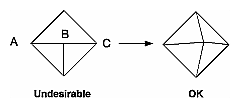Figure 2-13 : Modifying an Undesirable T-intersection

If you're constructing a closed surface, make sure to use exactly the same numbers for coordinates at the beginning and end of a closed loop, or you can get gaps and cracks due to numerical round-off. Here's a two-dimensional example of bad code:
```/* don't use this code */

#define PI 3.14159265
#define EDGES 30

/* draw a circle */
for (i = 0; i < EDGES; i++) {
glBegin(GL_LINE_STRIP);
glVertex2f(cos((2*PI*i)/EDGES), sin((2*PI*i)/EDGES);
glVertex2f(cos((2*PI*(i+1))/EDGES),
sin((2*PI*(i+1))/EDGES);
glEnd();
}```
The edges meet exactly only if your machine manages to calculate the sine and cosine of 0 and of (2*PI*EDGES/EDGES) and gets exactly the same values. If you trust the floating-point unit on your machine to do this right, the authors have a bridge they'd like to sell you.... To correct the code, make sure that when i == EDGES-1, you use 0 for the sine and cosine, not 2*PI*EDGES/EDGES.

Finally, note that unless tessellation is very fine, any change is likely to be visible. In some animations, these changes are more visually disturbing than the artifacts of undertessellation.

### An Example: Building an Icosahedron

To illustrate some of the considerations that arise in approximating a surface, let's look at some example code sequences. This code concerns the vertices of a regular icosahedron (which is a Platonic solid composed of twenty faces that span twelve vertices, each face of which is an equilateral triangle). An icosahedron can be considered a rough approximation for a sphere. Example 2-4 defines the vertices and triangles making up an icosahedron and then draws the icosahedron.

Example 2-4 : Drawing an Icosahedron

```#define X .525731112119133606
#define Z .850650808352039932

static GLfloat vdata = {
{-X, 0.0, Z}, {X, 0.0, Z}, {-X, 0.0, -Z}, {X, 0.0, -Z},
{0.0, Z, X}, {0.0, Z, -X}, {0.0, -Z, X}, {0.0, -Z, -X},
{Z, X, 0.0}, {-Z, X, 0.0}, {Z, -X, 0.0}, {-Z, -X, 0.0}
};

static GLint tindices = {
{0,4,1}, {0,9,4}, {9,5,4}, {4,5,8}, {4,8,1},
{8,10,1}, {8,3,10}, {5,3,8}, {5,2,3}, {2,7,3},
{7,10,3}, {7,6,10}, {7,11,6}, {11,0,6}, {0,1,6},
{6,1,10}, {9,0,11}, {9,11,2}, {9,2,5}, {7,2,11} };

for (i = 0; i < 20; i++) {
/* color information here */
glBegin(GL_TRIANGLE);
glVertex3fv(&vdata[tindices[i]]);
glVertex3fv(&vdata[tindices[i]]);
glVertex3fv(&vdata[tindices[i]]);
glEnd();
}```
The strange numbers X and Z are chosen so that the distance from the origin to any of the vertices of the icosahedron is 1.0. The coordinates of the twelve vertices are given in the array vdata[][], where the zeroth vertex is {-X, 0.0, Z}, the first is {X, 0.0, Z}, and so on. The array tindices[][] tells how to link the vertices to make triangles. For example, the first triangle is made from the zeroth, fourth, and first vertex. If you take the vertices for triangles in the order given, all the triangles have the same orientation.

The line that mentions color information should be replaced by a command that sets the color of the ith face. If no code appears here, all faces are drawn in the same color, and it'll be impossible to discern the three-dimensional quality of the object. An alternative to explicitly specifying colors is to define surface normals and use lighting, as described in the next section.

In all the examples described in this section, unless the surface is to be drawn only once, you should probably save the calculated vertex and normal coordinates so that the calculations don't need to be repeated each time that the surface is drawn. This can be done using your own data structures or by constructing display lists (see Chapter 4 .)

#### Defining the Icosahedron's Normals

If the icosahedron is to be lit, you need to supply the vector normal to the surface. With the flat surfaces of an icosahedron, all three vertices defining a surface have the same normal vector. Thus, the normal needs to be specified only once for each set of three vertices. The code in Example 2-5 can replace the "color information here" line in Example 2-4 for drawing the icosahedron.

Example 2-5 : Supplying Normals for an Icosahedron

```GLfloat d1, d2, norm;
for (j = 0; j < 3; j++) {
d1[j] = vdata[tindices[i]][j] - vdata[tindices[i]][j];
d2[j] = vdata[tindices[i]][j] - vdata[tindices[i]][j];
}
normcrossprod(d1, d2, norm);
glNormal3fv(norm);```
The function normcrossprod() produces the normalized cross product of two vectors, as shown in Example 2-6 .

Example 2-6 : Calculating the Normalized Cross Product of Two Vectors

```void normalize(float v) {
GLfloat d = sqrt(v*v+v*v+v*v);
if (d == 0.0) {
error("zero length vector");
return;
}
v /= d; v /= d; v /= d;
}

void normcrossprod(float v1, float v2, float out)
{
GLint i, j;
GLfloat length;

out = v1*v2 - v1*v2;
out = v1*v2 - v1*v2;
out = v1*v2 - v1*v2;
normalize(out);
}```
If you're using an icosahedron as an approximation for a shaded sphere, you'll want to use normal vectors that are perpendicular to the true surface of the sphere, rather than being perpendicular to the faces. For a sphere, the normal vectors are simple; each points in the same direction as the vector from the origin to the corresponding vertex. Since the icosahedron vertex data is for an icosahedron of radius 1, the normal and vertex data is identical. Here is the code that would draw an icosahedral approximation of a smoothly shaded sphere (assuming that lighting is enabled, as described in Chapter 6 ):
```for (i = 0; i < 20; i++) {
glBegin(GL_POLYGON);
glNormal3fv(&vdata[tindices[i]]);
glVertex3fv(&vdata[tindices[i]]);
glNormal3fv(&vdata[tindices[i]]);
glVertex3fv(&vdata[tindices[i]]);
glNormal3fv(&vdata[tindices[i]]);
glVertex3fv(&vdata[tindices[i]]);
glEnd();
}```

#### Improving the Model

A twenty-sided approximation to a sphere doesn't look good unless the image of the sphere on the screen is quite small, but there's an easy way to increase the accuracy of the approximation. Imagine the icosahedron inscribed in a sphere, and subdivide the triangles as shown in Figure 2-14 . The newly introduced vertices lie slightly inside the sphere, so push them to the surface by normalizing them (dividing them by a factor to make them have length 1). This subdivision process can be repeated for arbitrary accuracy. The three objects shown in Figure 2-14 use twenty, eighty, and three hundred and twenty approximating triangles, respectively.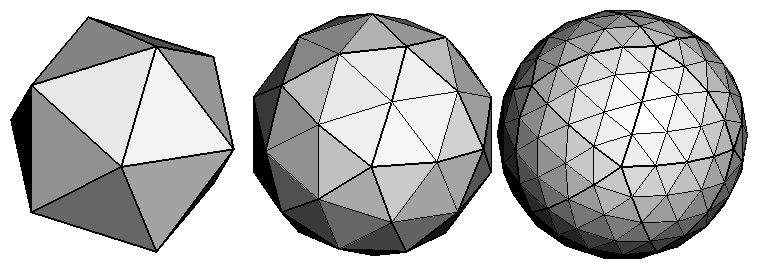Figure 2-14 : Subdividing to Improve a Polygonal Approximation to a Surface

Example 2-7 performs a single subdivision, creating an eighty-sided spherical approximation.

Example 2-7 : Single Subdivision

```void drawtriangle(float *v1, float *v2, float *v3)
{
glBegin(GL_POLYGON);
glNormal3fv(v1); vlVertex3fv(v1);
glNormal3fv(v2); vlVertex3fv(v2);
glNormal3fv(v3); vlVertex3fv(v3);
glEnd();
}

void subdivide(float *v1, float *v2, float *v3)
{
GLfloat v12, v23, v31;
GLint i;

for (i = 0; i < 3; i++) {
v12[i] = v1[i]+v2[i];
v23[i] = v2[i]+v3[i];
v31[i] = v3[i]+v1[i];
}
normalize(v12);
normalize(v23);
normalize(v31);
drawtriangle(v1, v12, v31);
drawtriangle(v2, v23, v12);
drawtriangle(v3, v31, v23);
drawtriangle(v12, v23, v31);
}

for (i = 0; i < 20; i++) {
subdivide(&vdata[tindices[i]],
&vdata[tindices[i]],
&vdata[tindices[i]]);
}```
Example 2-8 is a slight modification of Example 2-7 that recursively subdivides the triangles to the proper depth. If the depth value is 0, no subdivisions are performed, and the triangle is drawn as is. If the depth is 1, a single subdivison is performed, and so on.

Example 2-8 : Recursive Subdivision

```void subdivide(float *v1, float *v2, float *v3, long depth)
{
GLfloat v12, v23, v31;
GLint i;

if (depth == 0) {
drawtriangle(v1, v2, v3);
return;
}
for (i = 0; i < 3; i++) {
v12[i] = v1[i]+v2[i];
v23[i] = v2[i]+v3[i];
v31[i] = v3[i]+v1[i];
}
normalize(v12);
normalize(v23);
normalize(v31);
subdivide(v1, v12, v31, depth-1);
subdivide(v2, v23, v12, depth-1);
subdivide(v3, v31, v23, depth-1);
subdivide(v12, v23, v31, depth-1);
}```

#### Generalized Subdivision

A recursive subdivision technique such as the one described in Example 2-8 can be used for other types of surfaces. Typically, the recursion ends either if a certain depth is reached, or if some condition on the curvature is satisfied (highly curved parts of surfaces look better with more subdivision).

To look at a more general solution to the problem of subdivision, consider an arbitrary surface parameterized by two variables u and u. Suppose that two routines are provided:

```void surf(GLfloat u, GLfloat vertex, GLfloat normal);
float curv(GLfloat u);```
If surf() is passed u[], the corresponding three-dimensional vertex and normal vectors (of length 1) are returned. If u[] is passed to curv(), the curvature of the surface at that point is calculated and returned. (See an introductory textbook on differential geometry for more information about measuring surface curvature.)

Example 2-9 shows the recursive routine that subdivides a triangle either until the maximum depth is reached or until the maximum curvature at the three vertices is less than some cutoff.

Example 2-9 : Generalized Subdivision

```void subdivide(float u1, float u2, float u3,
float cutoff, long depth)
{
GLfloat v1, v2, v3, n1, n2, n3;
GLfloat u12, u23, u32;
GLint i;

if (depth == maxdepth || (curv(u1) < cutoff &&
curv(u2) < cutoff && curv(u3) < cutoff)) {
surf(u1, v1, n1); surf(u2, v2, n2); surf(u3, v3, n3);
glBegin(GL_POLYGON);
glNormal3fv(n1); glVertex3fv(v1);
glNormal3fv(n2); glVertex3fv(v2);
glNormal3fv(n3); glVertex3fv(v3);
glEnd();
return;
}
for (i = 0; i < 2; i++) {
u12[i] = (u1[i] + u2[i])/2.0;
u23[i] = (u2[i] + u3[i])/2.0;
u31[i] = (u3[i] + u1[i])/2.0;
}
subdivide(u1, u12, u31, cutoff, depth+1);
subdivide(u2, u23, u12, cutoff, depth+1);
subdivide(u3, u31, u23, cutoff, depth+1);
subdivide(u12, u23, u31, cutoff, depth+1);
}```

[Previous chapter] [Next chapter]
See the About page for copyright, authoring and distribution information.